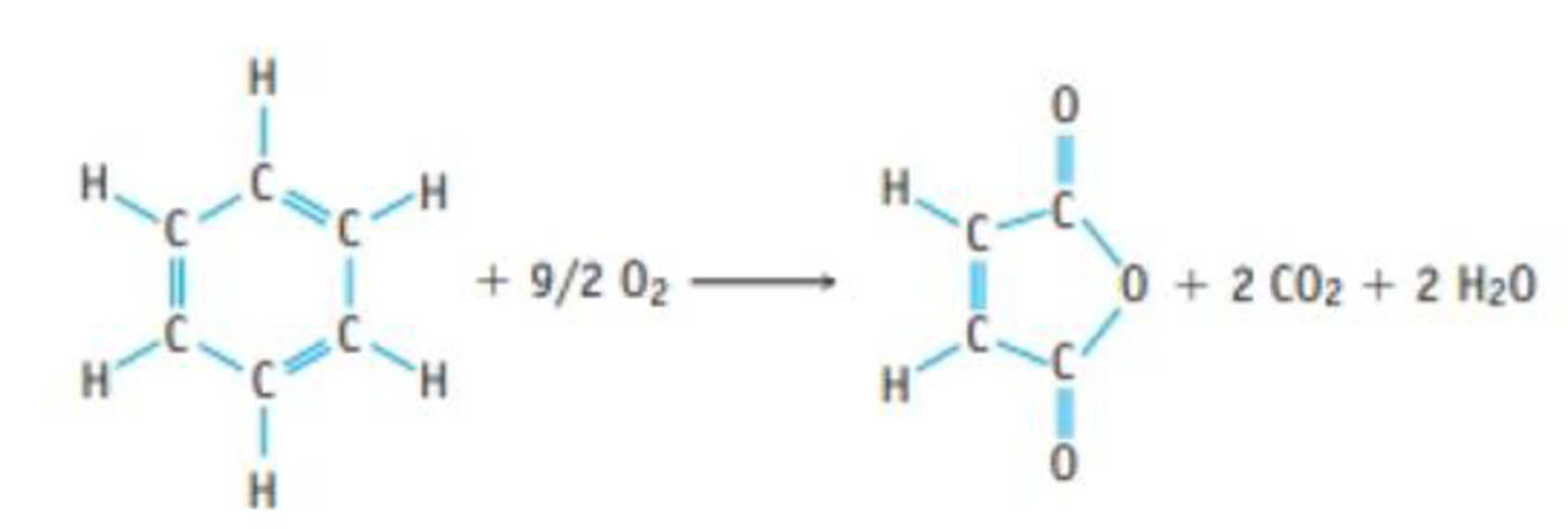Chapter 4, Problem 141SCQ

Chapter
Section
Textbook Problem

ATOM ECONOMY: Benzene, C6H6, is a common compound, and it can be oxidized to give maleic anhydride, C4H2O3, which is used in turn to make other important compounds.(a) What is the % atom economy for the synthesis of maleic anhydride from benzene by this reaction? (b) If 972 g of maleic anhydride is produced from exactly 1.00 kg of benzene, what is the percent yield of the anhydride? What mass of the by-product CO2 is also produced?

(a)

Interpretation Introduction

Interpretation:

The % atom economy for the product in the given reaction has to be calculated.

Concept introduction:

• Atom economy is one of the key parameter used to evaluate the efficiency of a reaction.

%atomeconomy=molarmassofatomutilizedmolarmassofreactants×100%

• Percent yield of reaction is the ratio of mass of actual yield to the mass of theoretical yield and multiplied with hundred.
• Numberofmole=GivenmassofthesubstanceMolarmass
• The molar mass of an element or compound is the mass in grams of 1 mole of that substance, and it is expressed in the unit of grams per mol (g/mol).
• For chemical reaction balanced chemical reaction equation written in accordance with the Law of conservation of mass.
• Law of conservation of mass states that for a reaction total mass of the reactant and product must be equal.
• Stoichiometric factor is a relationship between reactant and product which is obtained from the balanced chemical equation for a particular reaction.
Explanation

Atom economy is one of the key parameter used to evaluate the efficiency of a reaction. Atom economy percentage of a reaction can be calculated by using the following equation.

%atomeconomy=molarmassofatomutilizedmolarmassofreactants×100%

The balanced equation for the synthesis of maleic anhydride from benzene is,

In the above reaction reactant molecule contains 6C,6H,1O,and92O2 so the combined molar mass of the reactants is 222g/mol

The product molecule maleicanhydrideC4H2O3 contains 4C

(b)

Interpretation Introduction

Interpretation:

The percentage yield and the mass of CO2 produced in the reaction also has to be calculated.

Concept introduction:

• Atom economy is one of the key parameter used to evaluate the efficiency of a reaction.

%atomeconomy=molarmassofatomutilizedmolarmassofreactants×100%

• Percent yield of reaction is the ratio of mass of actual yield to the mass of theoretical yield and multiplied with hundred.
• Numberofmole=GivenmassofthesubstanceMolarmass
• The molar mass of an element or compound is the mass in grams of 1 mole of that substance, and it is expressed in the unit of grams per mol (g/mol).
• For chemical reaction balanced chemical reaction equation written in accordance with the Law of conservation of mass.
• Law of conservation of mass states that for a reaction total mass of the reactant and product must be equal.
• Stoichiometric factor is a relationship between reactant and product which is obtained from the balanced chemical equation for a particular reaction.

Still sussing out bartleby?

Check out a sample textbook solution.

See a sample solution

The Solution to Your Study Problems

Bartleby provides explanations to thousands of textbook problems written by our experts, many with advanced degrees!

Get Started